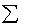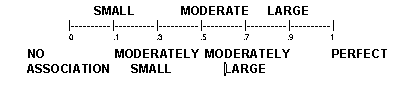previous lesson

Theta

ASSOCIATION: Bivariate Ordinal/Nominal Distribution

Theta (): =Di/T2

Where: T2 = Sum of product of nominal totals multiplied by each other nominal total, taking them 2 at a time.
For instance, if the totals are 3, 4, 7, 2: T2 = 3x4 + 3x7 + 3x2 + 4x7 + 4x2 + 7x2 = 89.

Di = | Ai - Bi |

Where: Ai = For any pair of nominal categories, take the frequency in each cell of the first category and multiply it by the sum of the frequencies in the cells to the right of it in the second nominal category. Sum these products.

Bi = For any pair of nominal categories, take the frequency in each cell of the first category and multiply it by the sum of the frequencies in the cells to the left of it in the second nominal category. Sum these products.
*It is crucial that table is set up so that nominal categories are on the side and ordinal categories are across the top.

Example:

 Number of job offers Major 20-24 18-19 17 16 13-15 Criminal Justice 10 10 2 1 1 24 Public Administration 1 2 10 10 1 24 Child Development 1 1 2 2 10 16 12 13 14 13 12 64

Q: What is the association between major and job offers?
A: CJ = criminal justice
CD = child development

Di (CJ,PA) = Ai | [10(2+10+10+1) + 10(10+10+1) + 2(10+1) + 1(1)]
- Bi[1(1+2+10+10) + 1(1+2+10) + 2(1+2) + 10(1)] | = 411

Di (CJ,CD) = Ai | [10(1+2+2+10) + 10(2+2+10) + 2(2+10) + 1(10)]
- Bi[1(1+1+2+2) + 1(1+1+2) + 2(1+1) + 10(1)] | = 300

Di (PA,CD) = Ai | [1(1+2+2+10) + 2(2+2+10) + 10(2+10) + 10(10)]
- Bi[1(1+1+2+2) + 10(1+1+2) + 10(1+1) + 2(1)] | = 195

Theta (): = (411 +300 +195) / [24(24) + 24(16) + 24(16)] = .67

Interpretation: Use scale, always positiveor Change to percent: "In % of the comparisons made, (nominal variable) showed systematic differences in (ordinal variable).

From example above:
.67 = "A moderately large association between major and number of job offers." Or
"In 67% of the comparisons made, persons with different majors showed systematic differences in their number of job offers.